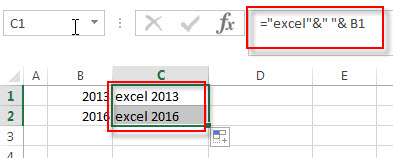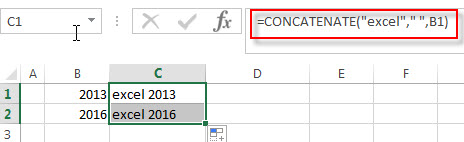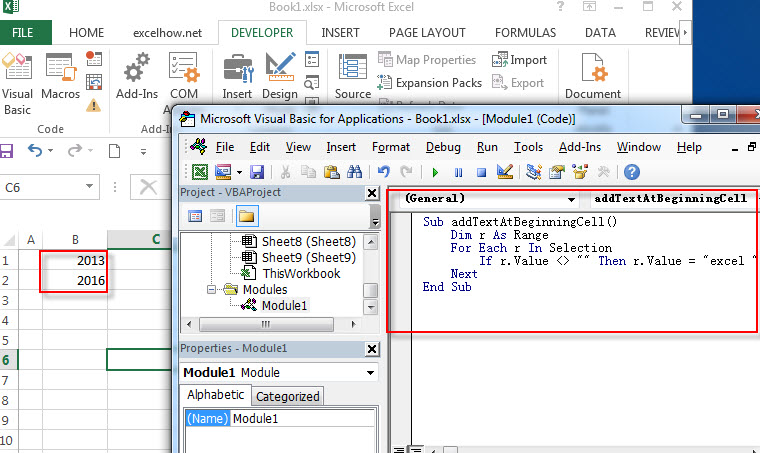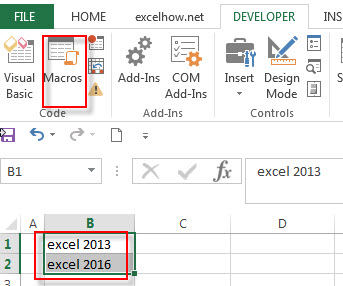How to add text to the beginning of all cells

This post explains that how to add the specified text or characters to the beginning of all cells in excel. How to create an excel formula to add same text string or characters to the beginning of text string in one Cell. How to create an excel macro to add specific text to the beginning of the text in all of cells.

Add text to the beginning of all cells with Formula

If you want to add the specific text or characters into the beginning of the text in one cell or all cells, you can create an excel formula based on the concatenate operator or CONCATENATE function.

Assuming that you want to add text “excel” into the beginning of the text in Cell B1, you can write down the following formula:

="excel"&" "& B2

OR

=CONCATENATE(“excel”,””,B1)

You can enter the above formulas in Cell C1, and then drag the fill handle down to other cells in column C and you will see that the specific text will add the beginning of the text in Cell B1.Add text to the beginning of all cells with Excel VBA

You can create a new excel macro to add text string “excel” to the beginning of text in Cell B1 in Excel VBA, just refer to the below steps:

1# click on “Visual Basic” command under DEVELOPER Tab.2# then the “Visual Basic Editor” window will appear.

3# click “Insert” ->”Module” to create a new module4# paste the below VBA code into the code window. Then clicking “Save” button.

Dim r As Range
For Each r In Selection
If r.Value <> "" Then r.Value = "excel " & r.Value
Next
End Sub5# back to the current worksheet, then run the above excel macro, you will see that the specific text “excel” has been added into the beginning of the text in all selected Cells.Related Functions

• Excel Concat function
The excel CONCAT function combines 2 or more strings or ranges together.This is a new function in Excel 2016 and it replaces the CONCATENATE function.The syntax of the CONCAT function is as below:=CONCAT (text1,[text2],…)…
Related Posts

VBA Macro For VLOOKUP From Another Sheet

In the previous post, you should know that how to fix or remove the #N/A error when using VLOOKUP formula to lookup value from another sheet. And this post will show you how to use VBA code to vlookup data ...

How To Insert Comments in Protected Worksheet in Excel

This post will show you how to allow comments in a protected worksheet in Excel. You can easily to insert comments into cells in a normal worksheet in Excel, but if want to insert a comment in a worksheet that ...

How To Convert Text to Upper Cases(Using VBA) in Excel

This post will show you how to switch from lower case to upper case in Excel. and I am going to show you two different ways of converting text to upper cases using formula or VBA macro in Excel 2013,Excel ...

How To Hide Every Other Row in Excel (Using VBA)

This post will show you how to hide alternate rows or columns in Excel or how to hide every third, fourth, fifth row or column in Excel. If you want to hide every other row in your current worksheet, how ...

How to Disable the Save As Prompt in Excel

This post will show you how to use a VBA Macro to save an Excel file and overwrite any existing file without a prompt so that you are going to get the little window that says file already exists do ...

How to Count Cells that Contain even or odd numbers in Excel

This post will guide you how to count the number of cells that contain odd or even numbers within a range of cells using a formula in Excel 2013/2016.How do I count cells that contain odd numbers through the use ...

How to Count Cells that Contain negative Numbers in Excel

This post will guide you how to count the number of cells that contain negative numbers within a range of cells using a formula in Excel 2013/2016.You can count the number of negative numbers in your data using easy functions ...

How to Count Cells Are Not Blank or Empty in Excel

This post will guide you how to count cells that are not blank or empty in a given range cells using a formula in Excel 2013/2016.How do I count the number of cells that are not blank in a particular ...

How to Count Cells Not equal to X or Y in Excel

This post will guide you how to count the number of cells not equal to criteria X or Y in a given range cells using a formula in Excel 2013/2016.You can easily to count cells equal to or not equal ...

How to Count Cells Less Than a Specific Value in Excel

This post will guide you how to count the number of cells less than a particular numeric value in a given range cells using a formula in Excel 2013/2016. How do I count cells that are less than a specific ...

Sidebar